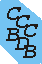Computational Chemistry Comparison and Benchmark DataBase Release () Standard Reference Database 101 National Institute of Standards and Technology Home All data for one species Geometry Experimental Calculated Comparisons Bad Calculations Tutorials and Explanations Vibrations Experimental Calculated Scale factors Reactions Entropies Ions List Ions Energy Electron Affinity Proton Affinity Ionization changes point group Experimental One molecule all properties One property a few molecules Geometry Vibrations Energy Electrostatics Reference Data Calculated Energy Optimized Reaction Internal Rotation Orbital Nuclear repulsion energy Correlation Ion Excited State Basis Set Extrapolation Geometry Vibrations Frequencies Zero point energy (ZPE) Scale Factors Bad Calculations Electrostatics Charges Dipole Quadrupole Polarizability Spin Entropy and Heat Capacity Reaction Lookup by property Comparisons Geometry Vibrations Energy Entropy Electrostatics Ion Resources Info on Results Calculations Done Basis functions used I/O files Glossary Conversion Forms Links NIST Links External links Thermochemistry Tutorials Vibrations Entropy Energy Electrostatics Geometry Cost Bad Calculations FAQ Help Units Choose Units Explanations Credits Just show me Summary Using List Recent molecules Molecules Geometry Vibrations Energy Similar molecules Ions, Dipoles, etc. Index of CCCBDB Feedback You are here: Resources > Tutorials > Vibrations > Zero-point energies

# Vibrational Zero-Point Energies (ZPE)

The vibrational zero-point energy is the energy difference between the lowest point on the potential energy surface (equilibrium energy) and the energy of the vibrationless energy level (v=0). It is not possible to measure the ZPE. The ZPE can be approximated as half the fundamental vibrational frequencies. This assumes the vibrational frequencies are harmonic and there are no vibration-rotation interactions. Given more vibrational and rotational details, such as anharmonic contants (ωexe, ωeye, ...), vibration-ration interaction constants (αe) better approximations to the ZPE can be made.

For diatomics we use:See the paper by Irikura: K. K. Irikura "Experimental Vibrational Zero-Point Energies: Diatomic Molecules" J. Phys. Chem. Ref. Data 36(2), 389, 2007